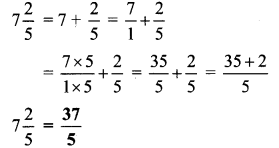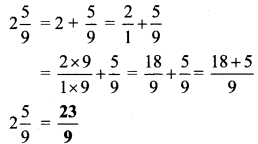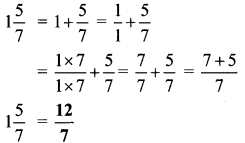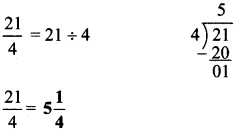# Maharashtra Board Class 6 Maths Solutions Chapter 4 Operations on Fractions Practice Set 9

## Maharashtra State Board Class 6 Maths Solutions Chapter 4 Operations on Fractions Practice Set 9

Question 1.
Convert into improper fractions:
i. $$7 \frac{2}{5}$$
ii. $$5 \frac{1}{6}$$
iii. $$4 \frac{3}{4}$$
iv. $$2 \frac{5}{9}$$
v. $$1 \frac{5}{7}$$
Solution:
i. $$7 \frac{2}{5}$$ii. $$5 \frac{1}{6}$$iii. $$4 \frac{3}{4}$$iv. $$2 \frac{5}{9}$$v. $$1 \frac{5}{7}$$Question 2.
Convert into mixed numbers:
i. $$\frac { 30 }{ 7 }$$
ii. $$\frac { 7 }{ 4 }$$
iii. $$\frac { 15 }{ 12 }$$
iv. $$\frac { 11 }{ 8 }$$
v. $$\frac { 21 }{ 4 }$$
v. $$\frac { 20 }{ 7 }$$
Solution:
i. $$\frac { 30 }{ 7 }$$ii. $$\frac { 7 }{ 4 }$$iii. $$\frac { 15 }{ 12 }$$iv. $$\frac { 11 }{ 8 }$$v. $$\frac { 21 }{ 4 }$$v. $$\frac { 20 }{ 7 }$$Question 3.
Write the following examples using fraction:
i. If 9 kg rice is shared among 5 people, how many kilograms of rice does each person get?
ii. To make 5 shirts of the same size, 11 meters of cloth is needed. How much cloth is needed for one shirt?
Solution:
i. Total quantity of rice = 9 kg
Number of people = 5
∴ Kilograms of rice received by each person = $$\frac { 9 }{ 5 }$$
∴ Each person will get $$\frac { 9 }{ 5 }$$ kg of rice.

ii. Total meters of cloth = 11 meters
Number of shirts to be made = 5
Meters of cloth needed to make 1 shirt = $$\frac { 11 }{ 5 }$$
∴ Cloth needed to make 1 shirt is $$\frac { 11 }{ 5 }$$ meters.